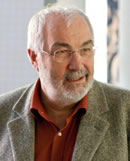Home NEMO 2014 Lectures Conceptual Modelling: The Role of Formal Calculi

## Conceptual Modelling: The Role of Formal CalculiProf. Dr. Wolfgang Reisig

Humboldt University of Berlin, Germany

Modelling Methods are frequently classified as informal, semiformal or formal, respectively. Each of the three classes has its particular advantages. In this contribution we focus on formal methods, and survey their common mathematical basis. Hence, instead of discussing subtle differences of models, we show what they have in common. In detail we separate three aspects: -Behavioural models: automata, transition systems, MSC, scenarios, finite and infinite behaviour, inductive invariants, simulation and bisimulation, process algebras, Petri nets, BPMN. -Data depicting models: signatures, sigma structures, terms, quantors, first order logic, ASM, Z, high level Petri nets. -Properties of models: composition and refinement, temporal logic, model checking. Participants at this course will be able to understand the essentials of the many variants of modern techniques and corresponding tool based analysis methods for enterprise modelling.

Lecture at NEMO2014

Date/Time: Monday, July 14, 2014 at 09:00